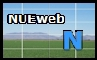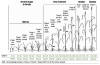Nitrogen Use Efficiency DefinitionsImportance of "YIELD" for estimating Nitrogen Use Efficiency
Nitrogen use efficiency and/or fertilizer recovery in crop production systems can be computed using a wide range of methods.  Those specifically designated for crop production systems follow.  In addition, nitrogen use efficiency has been estimated using world N consumption and crop production, but on macro scales (Agron. J. 91:357-363). Other methods (Difference Method, Isotopic Method (Enriched and Depleted)) are delineated below.  The components of Nitrogen Use Efficiency (NUE) are further discussed, as initially reported by Moll et al. (1982).  Regardless of the method, almost all result in estimated NUE for cereal production that average between 30 and 35%.  Topdress or sidedress N applications in the middle of the season can result in greater NUE's (>50%).  Because the risk of N loss is greater when N is applied in the fall, N should be applied in the spring to minimize risk and optimize profitability regardless of tillage system (Vetsch and Randall, 2004).

Keeney 1982 Justification, (reference used in AJ 91:357)
1. Calculating N Use Efficiency using The Difference Method

Difference method

PFR = (NF)-(NC) / R

NF = total crop N uptake (corn, wheat, rice, etc.) from fertilized plots
NC = total crop N uptake (corn, wheat, rice, etc.) from unfertilized plots
R = rate of fertilizer N applied
PFR = percent fertilizer recovery

EXAMPLE below:

 Applied N Grain Yield Grain N N uptake Fertilizer Recovery kg/ha kg/ha % kg/ha (NUE), % 0 1000 2 20 - 50 1300 2.1 27.3 (27.3-20)/50=14.6 100 2000 2.2 44 (44-20)/100=24 150 2000 2.3 46 (46-20)/150=17

2. Macro Calculation of World NUE (method defined)

3. Moll et al. (1982), components of NUE
resence of two primary components of N use efficiency:
(1)Efficiency of absorption or uptake (Nt/Ns)
(2)Efficiency with which N absorbed is utilized to produce grain (Gw/Nt)
Nt = total N in the plant at maturity (grain + stover)
Ns = nitrogen supply or rate of fertilizer N
Gw = grain weight (all expressed in the same units)

Consideration of additional parameters not discussed in Moll et al. (1982) *plant N loss

• Maximum N accumulation has been found to occur at or near flowering in wheat and corn and not at harvest.
• In order to estimate plant N loss without the use of labeled N forms, the stage of growth where maximum N accumulation is known to occur needs to be identified.
• The amount of N remaining in the grain + straw or stover, is subtracted from the amount at maximum N accumulation to estimate potential plant N loss (difference method).
• Use of difference methods for estimating plant N loss are flawed since continued uptake is known to take place beyond flowering or the point of maximum N accumulation.
Davis, R.L., J.J. Patton, R.K. Teal, Y. Tang, M.T. Humphreys, J. Mosali, K. Girma, J.W. Lawles, S.M. Moges, A. Malapati, J.Si, H. Zhang, S. Deng, G.V. Johnson, R.W. Mullen, and W.R. Raun. 2003. Nitrogen balance in the Magruder Plots following 109 years in continuous winter wheat. J. Plant Nutr. 26(8):1561-1580.

COMPONENTS of NUE (Moll et al., 1982), graphic illustration
4. Nitrogen Use Efficiency (Recovery) Using Isotopic Methods

Enriched 15N:

Materials with a greater than natural concentration of 15N % plant N derived from fertilizer = %15N excess in sample  % 15N excess in fertilizer

Depleted 15N:

Materials with a lower than natural concentration of 15N (0.003 - 0.01 atom % 15N) or (< 0.01 atom % 15N)

Use of isotopic 14N

Studies involving residual soil nitrogen are not practical with depleted materials due to the high dilution factor

% plant N derived from the fertilizer =

(Nu - Nt)/(Nu - (Nf/n))
Nu =atom % 15N in unfertilized plants
Nt = atom % 15N in fertilized plants
Nf = atom % 15N in the fertilizer (for example 0.006%)
n = the plant discrimination factor between 14N and 15N.

If it is assumed that there is no discrimination between 14N and 15N, then n = 1.

5. Fertilizer N Recovery (Varvel and Peterson, 1990)

1. Difference method

PFR = (NF)-(NC) / R

NF = total N uptake in corn from N fertilized plots
NC = total N uptake in corn from unfertilized plots
R = rate of fertilizer N applied
PFR = percent fertilizer recovery

2. Isotopic method (Depleted material)

PFR = ((NF) x (C-B)/D) / R

NF = total N uptake in corn from N fertilized plots
B = atom % 15N of plant tissue from N fertilized plots
C = atom % 15N of plant tissue from unfertilized plots (0.366%)
D = depleted atom % 15N in applied N fertilizer
R = rate of applied 15N-labeled fertilizer

3. Isotopic method (Enriched material, Sanchez et al., 1987)

F = As-Ar/Af-Ar
F= fraction of total N uptake derived from 15N enriched fertilizer
As = atom % 15N measured in the harvested plant sample
Af = atom % 15N in the enriched fertilizer
Ar = atom % 15N of the reference harvested plant material from non 15N enriched fertilizer treatments

Ef = F x total N uptake
Ef = uptake of 15N enriched fertilizer

Shearer and Legg (1975) found that d15N of wheat plants decreased as the N application rate increased.

d15N = atom % 15N (sample) - atom % 15N (standard) x 1000 / atom % 15 N (standard)

15N composition of the total N of grain and leaf samples of corn (Zea mays L.) decreased systematically as N fertilizer rates increased (Kohl et al., 1973). This result was considered to be consistent with increasing contributions of fertilizer N to plants as the rate of applied N increased.

Hauck and Bremner, 1976

percent nitrogen recovered (plant or soil) =

= 100P (c-b) / f(a-b)

P = total N in the plant part or soil in kg ha-1
f = rate of 15N fertilizer applied
a = atom percent 15N in the labeled fertilizer
b = atom percent 15N in the plant part or soil receiving no 15N
c = atom percent 15N in the plant part or soil that did receive 15N

unlabeled N uptake = (total N uptake in grain and straw) -

[N rate(% recovery of 15N in grain and straw)]

6. Fertilizer N Recovery, Mass Balance (Davis et al., 2003)

The Magruder Plots are the oldest continuous soil fertility wheat research plots in the Great Plains region, and are one of the oldest continuous soil fertility wheat plots in the world.

They were initiated in 1892 by Alexander C. Magruder who was interested in the productivity of native prairie soils when sown continuously to winter wheat.

Simple estimate of nitrogen (N) balance
1. account for N applied,
2. account for N removed in the grain
3. account for estimated plant N loss, denitrification, nitrate leaching
4. account for non-symbiotic N fixation, N applied in the rainfall,
5. have an estimate of total soil N (0-30 cm) at the beginning of the experiment
6. have an estimate of total soil N (0-30 cm) at the end of the experiment

Manure plots: total soil N decreased from 6890 kg N ha-1 in the surface 0-30 cm in 1892, to 3198 kg N ha-1 in 2002.

Check plots (no nutrients applied for 109 years) only 2411 kg N ha-1 or 35% of the original total soil organic N remains.

Nitrogen removed in the grain averaged 38.4 kg N ha-1yr-1
N additions (manure, N in rainfall, N via symbiotic N fixation) averaged 44.5 kg N ha-1 yr-1 in the Manure plots.

Following 109 years, unaccounted N ranged from 229 to 1395 kg N ha-1.
By year basis, translates into 2 to 13 kg N ha-1yr-1 that were unaccounted
Manure plots, estimate of nitrogen use efficiency (NUE) (N removed in the grain, minus N removed in the grain of the check plots, divided by the rate of N applied) was 32.8%

Similar to the 33% NUE for world cereal production reported in 1999.

World Nitrogen Use Efficiency for Cereal Production is 33%
Agronomy Journal, 91:357-363

EMAG_17

References  (Papers showing that NUE is between 30 and 40%)

Olson, R.V., and C.W. Swallow. 1984. Fate of labeled nitrogen fertilizer applied to winter wheat for five years. Soil Sci. Soc. Am. J. 48:583-586.

NUE: 27-33%

Davis, R.L., J.J. Patton, R.K. Teal, Y. Tang, M.T. Humphreys, J. Mosali, K. Girma, J.W. Lawles, S.M. Moges, A. Malapati, J.Si, H. Zhang, S. Deng, G.V. Johnson, R.W. Mullen, and W.R. Raun. 2003. Nitrogen balance in the Magruder Plots following 109 years in continuous winter wheat. J. Plant Nutr. 26(8):1561-1580.

NUE: 32.8%

NUE: 33%
(maize, rice, barley, sorghum, millet, oat, and rye)

Moll, R.H., E.J. Kamprath, and W.A. Jackson. 1982. Analysis and interpretation of factors which contribute to efficiency to nitrogen utilization. Agron. J. 74:562-564.

Olson, R.V., and C.W. Swallow. 1984. Fate of labeled nitrogen fertilizer applied to winter wheat for five years. Soil Sci. Soc. Am. J. 48:583-586.

NUE: 27-33%

NUE: 17-50%

NUE: > 100%

Vetsch, Jeff, and Gyles Randall. 2004. Corn production as affected by nitrogen application timing and tillage. Agron. J. 96:502-509.

Aparent N Recovery (87% for spring, 45% for fall applied N)

Varvel, G.E., and T.A. Peterson. 1990. Nitrogen fertilizer recovery by corn in monoculture and rotation systems. Agron. J. 82:935 to 938.

Methods to Improve, and/or measure NUE

Raun, W.R., J.B. Solie, M.L. Stone, K.L. Martin, K.W. Freeman, R.W. Mullen, H. Zhang J.S. Schepers, and G.V. Johnson.  2005.  Optical sensor based algorithm for crop nitrogen fertilization.  Commun. Soil Sci. Plant Anal. 36:2759-2781.

Vose, P. 1980. Introduction to nuclear techniques in agronomy and plant biology. Oxford, UK: Pergamon, 391 pp.  Amazon ListingNUE Results, Different Methods Method Authors Crop Year NUE Site Years Difference Olson and Swallow, SSSAJ Winter Wheat 1984 27 - 33% 5 Mass Balance Davis et al., JPN Winter Wheat 2003 32.8 109 Global Data Raun and Johnson, AJ Cereals 1999 33 comp. 1996 NUE components Moll et al., AJ Maize 1981 NA 1 Difference Raun et al., AJ Winter Wheat 2002 17-50 4 Difference/MB Edmonds et al., JPN Cereals 2009 100 comp. 2008 Apparent N recovery Vetsch and Randall, AJ Maize 2004 66 to 90 3 15N and Difference Varvel and Peterson, AJ Maize 1990 43 to 58 4 15N and Difference Olson, SSSAJ Maize 1980 26 2 Difference Barbieri et al., AJ Maize 2008 32.2 3

Plant Nitrogen Loss as NH3

1. Increased plant N loss with increasing nitrogen applied in winter wheat observed with 15N.  J. Plant Nutr. 23:219-230. (Lees et al., 2000)

2. Post-anthesis nitrogen loss from corn. Agron. J. 85:659-663. (Francis et al., 1981)

3. Effect of nitrogen rate on plant nitrogen loss in winter wheat varieties.  Desta et al. (1997). J. of Plant Nutr. 20:389-404. (pdf)

4. Gaseous Nitrogen Loss from Soybean Foliage
NEW N Management Strategy for Corn and Wheat
(91:357-363)
NUE Flow Chart (OSU discussion)
Components of Nitrogen Use Efficiency (added discussion of the Moll et al. 1982) manuscriptNitrogen Cycle Page

Combined Feekes growth stages, and corresponding GDD's, OklahomaCOMPOSITE FILE, NUE (several methods to estimate NUE delineated)